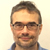# Equating heat loss to natural gas usage| Posted in Energy Efficiency and Durability on

I am trying to equate the heat loss from my house to equate with my natural gas usage.

I have a mid-1950s 11,385 sf ranch home in PA, zone 5. I performed a blower door test to measure my air loss, arriving at CFM50 rate of 1512 or an ACH50 rate of 7.97.

Going by the heat loss formula:

Q  =  V  x  ACH  x 0.0182  x  HDD  x  24

Where:
Q = Heat loss
V = Volume
ACH = Air changes per hour at 50 Pa
HDD = Heating Degree Days

Looking at January of 2019, we had 1012.4 HDD for my region.

This would mean

Q  =  11,385  x  7.97  x 0.0182  x  1012.4 x 24 = 40,126 kBtu

So for the month of January, I had 40,126 kBtu exfiltrate from my house.
Using Energy Star Tech Reference, there are 102.6 kBtu/ccf. This would mean I should be using about 391ccf of natural gas to maintain my 65 degree setpoint.

But I only used 100 ccf in January.

My initial thought was maybe this was due to furnace inefficiencies/unaccounted natural gas use (water heater, etc), but that would mean the ratio should be reversed: I should have used more natural gas than is exfiltrating from the house.

I actually did the heat loss calc for my natural gas consumption for the past two years, using excel, and found that over the winter months (Dec-Mar) the ratio is consistently around 4x what it should be. Accounting for water heater use and other odd variances, I am guessing there is a miscalc or missing variable that would lead it 4 times higher than it should be?

Anyone see what I’m missing?

(Thank in advance for any assistance)## Join the leading community of building science experts

### Replies

1.Expert Member
| | #1

The air infiltration, which is only part of the heat load. Conducted heat out of all the exterior surfaces of the house is usually a much larger number. The natural air infiltration rate is much MUCH smaller than when the house is at 50 pascals pressure relative to the outdoors, and estimating the ACH/natural from the ACH/50 measurement is extremely imprecise.

Also where is the correction for the equipment's combustion efficiency? Where is the 99% outside design temperature being factored in?

To run a load calculation based on fuel use, try this approach:

If you're maintaining the interior set point at 65F, use base 60F heating degree days for inferring the linear constant.

Also, can I assume the house size is actually 1,385 square feet, and not 11,385 square feet?

1.| | #4

The equation was pulled directly from Krigger's "Residential Energy: Cost Savings and Comfort for Existing Buildings" (2014) text. I might have misread the text...I think I was assuming that was giving me the total heat loss, but I see it was just the air infiltration.

Thank you for the article, I will definitely use that and see how it comes out.

The house dimensions are 23 x 33 ft, with 7 ft basement ceilings and 8 ft first floor ceilings. The entirety of both floors make up the conditioned space.

2.| | #2

I like to use Btu's per square foot per heating degree day as a heating energy use measurement. I have measured my own house this way for years and it will also show the increasing solar fraction as a slightly lower Btu/sf/hdd number as daylight increases. With your ACH50 number and the volume of the house you should be able to assign an infiltration heat loss number in Btu's.

3.| | #3

As Dana says, your formula has two problems:

a) it doesn't account for conduction losses
b) it assumes 50 pascals of pressure, which is way too high for the typical case

A more reasonable guess would be to 1/20 of your calculated value as the air leakage heat loss. Say 2Mbtu, leaving 8.28Mbtu due to conduction. Maybe 5.79 btu/sf/hdd (January, conduction only).

• |
• |
• |
• |